Students can download Maths Chapter 4 Geometry Ex 4.2 Questions and Answers, Notes, Samacheer Kalvi 9th Maths Guide Pdf helps you to revise the complete Tamilnadu State Board New Syllabus, helps students complete homework assignments and to score high marks in board exams.

## Tamilnadu Samacheer Kalvi 9th Maths Solutions Chapter 4 Geometry Ex 4.2

Question 1.
The angles of a quadrilateral are in the ratio 2 : 4 : 5 : 7. Find all the angles.
Solution:
Let the angles of a quadrilateral be 2x, 4x, 5x, and 7x.
Total angle of a quadrilateral = 360°
2x + 4x + 5x + 7x = 360°
18° = 360°
x = $$\frac{360°}{18}$$
= 20°
2x = 2 × 20° = 40°; 4x = 4 × 20° = 80°;
5x = 5 × 20° = 100°; 7x = 7 × 20° = 140°
The angles of a quadrilateral are 40°, 80°, 100° and 140°.Question 2.
In a quadrilateral ABCD, ∠A = 72° and ∠C is the supplementary of ∠A. The other two angles are 2x – 10 and x + 4. Find the value of x and the measure of all the angles.
Solution:∠A = 72°
∠C = 180° – 12° (∠A and ∠C are supplementary)
= 108°
∠A + ∠B + ∠C + ∠D = 360° (Total angles of quadrilateral)
72° + 2x – 10 + 108° + x + 4 = 360°
3x + 174° = 360°
x = $$\frac{186°}{3}$$
= 62°
The value of x is 62°
∠B = 2x – 10
= 2(62°) – 10
= 124° – 10°
= 114°
∠D = x + 4
= 62° + 4
= 66°
The other angles are 114°, 62° and 66°.Question 3.
ABCD is a rectangle whose diagonals AC and BD intersect at O. If ∠OAB = 46°, find ∠OBC.
Solution:
Since the diagonals of a rectangle AC and BD are equal and bisect each other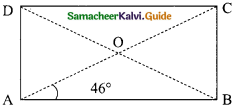∴ OA = OB
∠OAB = ∠OBA = 46°
Each angle of a rectangle measures 90°
∠ABC = 90°
∠ABO + ∠OBC = 90°
46° + ∠OBC = 90°
∠OBC = 90°-46°
∴ ∠OBC = 44°Question 4.
The lengths of the diagonals of a Rhombus are 12 cm and 16 cm. Find the side of the rhombus.
Solution:
Since the diagonals of a rhombus bisect each other at right angles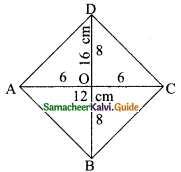AO = $$\frac{1}{2}$$AC = $$\frac{1}{2}$$ × 12 = 6 cm
BO = $$\frac{1}{2}$$BD = $$\frac{1}{2}$$ × 16 = 8 cm
In the right triangle AOD
= 6² + 8²
= 36 + 64
= 100
∴ AD = $$\sqrt{100}$$
= 10
∴ AB = BC = CD = AD = 10 cm.Question 5.
Show that the bisectors of angles of a parallelogram form a rectangle.
Solution:
Given: A parallelogram in which bisector of angle A, B, C, D intersect at P, Q, R, S to form a quadrilateral PQRS.
To prove: Quadrilateral PQRS is a rectangle.
Proof: Since ABCD is a parallelogram. Therefore, AB || DC.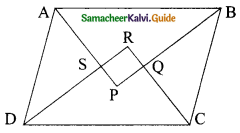Now, AB || DC, and transversal AD cuts them, so we have
∠A + ∠D = 180°
$$\frac{1}{2}$$∠A + $$\frac{1}{2}$$∠D = $$\frac{180°}{2}$$
But in ΔASD, we have
∠ADS + ∠DAS + ∠ASD = 180°
90° + ∠ASD = 180°
∠ASD = 90°
∠RSP = ∠ASD (vertically opposite angle)
∠RSP = 90°
Similarly, we can prove that
∠SRQ = 90°, ∠RQP = 90° and ∠QPS = 90°
Thus, PQRS is a quadrilateral each of whose angle is 90°.
Hence, PQRS is a rectangle.Question 6.
If a triangle and a parallelogram lie on the same base and between the same parallels then prove that the area of the triangle is equal to half of the area of parallelogram.
Solution:
Let ΔAPB and parallelogram ABCD lie on base AB and between parallels AB and PC.
To show area ΔAPB = $$\frac{1}{2}$$ Area (ABCD)
Now, draw BQ || AP. Then ABQP is a parallelogram.
Now area ABQP = Area ABCD
(They are on same base AB and between same parallels AB and PC)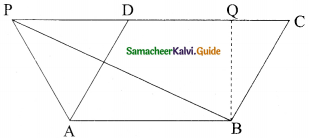⇒ ΔPAB ≅ ΔBQP
Area PAB = Area BQP
= $$\frac{1}{2}$$ Area ABQP
= $$\frac{1}{2}$$ Area ABCDQuestion 7.
Iron rods a, b, c, d, e, and f are making a design in a bridge as shown in the figure.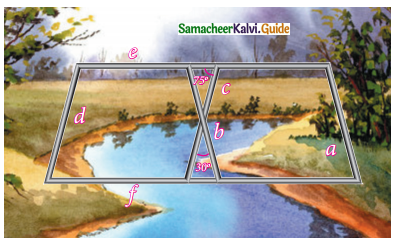If a || b, c || d, e || f, find the marked angles between
(i) b and c
(ii) d and e
(iii) d and f
(iv) c and f
Solution:
(i) Angle between b and c = 30°
(vertically opposite angles)

(ii) Angle between d and e = 180° – 75° = 105°
(sum of the adjacent angles of a parallelogram is 180°)

(iii) Angle between d and f = 75°
(opposite angles of a parallelogram)

(iv) Angle between c and f = 180° – 75° = 105°Question 8.
In the given figure, ∠A = 64°, ∠ABC = 58°. If BO and CO are the bisectors of ∠ABC and ∠ACB respectively of ΔABC, find x° and y°.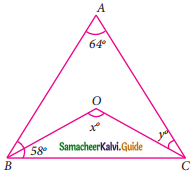Solution:
In the given ΔABC
∠A = 64° and ∠B = 58°
∠C = 180°- (64° + 58°)
= 180° – 122°
= 58°
Since OC is the bisector of ∠C
y = $$\frac{58°}{2}$$
= 29°
Given ΔOBC
∠OCB = $$\frac{58°}{2}$$ = 29°
∠OCB = 29°
∴ ∠BOC = 180°- (29° + 29°)
x = 180° – 58°
x = 122°
∠x = 122° and ∠y = 29°.Question 9.
In the given figure, if AB = 2, BC = 6, AE = 6, BF = 8, CE = 7 and CF = 7, compute the ratio of the area of quadrilateral ABDE to the area of ΔCDF.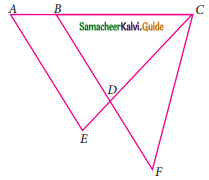Solution:
Given: AB = 2 cm, BC = 6 cm, AE = 6 cm, BF = 8 cm, CE = 7 cm and CF = 7 cm
Consider ΔAEC and ΔBCF.
In ΔAEC, AE = 6 cm, EC = 7 cm and AC = 8 cm (2 + 6 = 8)
In ΔBCF, BC = 6 cm, CF = 7 cm and BF = 8 cm
∴ ΔAEC s ΔBCF
∴ Area of ΔAEC = Area of ΔBCF (Two triangles are similar areas are equal)
Subtract area of ΔBDC on both sides we get,
Area of ΔAEC – Area of ΔBDC = Area of ΔBCF – Area of ΔBDC
Area of quadrilateral ABDE = Area of ΔCDFQuestion 10.
In the given figure, ABCD is a rectangle and EFGH is a parallelogram. Using the measurements given in the figure, what is the length ”d” of the segment that is perpendicular to $$\overline { HE }$$ and $$\overline { FG }$$?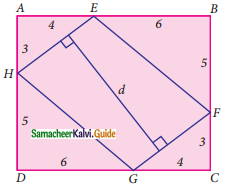Solution:
In the given figure ABCD is a rectangle and EFGH is a parallelogram.
In the right triangle AEH
HE = $$\sqrt{AH^{2} + AE^{2}}$$
= $$\sqrt{3^{2} + 4^{2}}$$
= $$\sqrt{9 + 16}$$
= $$\sqrt{25}$$
HE = 5
∴ GF = 5 (HE and Gf are opposite sides of a parallelogram)
In the right triangle
GC = $$\sqrt{GF^{2} – FC^{2}}$$
= $$\sqrt{5^{2} – 3^{2}}$$
= $$\sqrt{25 – 9}$$
= $$\sqrt{16}$$
∴ DG = 10 – 6 = 4
Area of ΔAEH + Area of ΔBEF + Area of ΔFCG + Area of ΔHDG
= $$\frac{1}{2}$$ × 3 × 4 + $$\frac{1}{2}$$ × 6 × 5 + $$\frac{1}{2}$$ × 3 × 4 + $$\frac{1}{2}$$ × 5 × 6
= (6 + 15 + 6 + 15)
= 42
∴ Area of 4 triangles = 42
Area of the parallelogram = Area of the rectangle ABCD – Area of 4 triangles.
= 10 × 8 – 42
= 80 – 42
= 38
b × h = 38
5 × d = 38
d = $$\frac{38}{5}$$
= 7$$\frac{3}{5}$$
Length of d = 7$$\frac{3}{5}$$ or 7.6Question 11.
In parallelogram ABCD of the accompanying diagram, line DP is drawn bisecting BC at N and meeting AB (extended) at P. From vertex C, line CQ is drawn bisecting side AD at M and meeting AB (extended) at Q. Lines DP and CQ meet at O. Show that the area of triangle QPO is $$\frac{9}{8}$$ of the area of the parallelogram ABCD.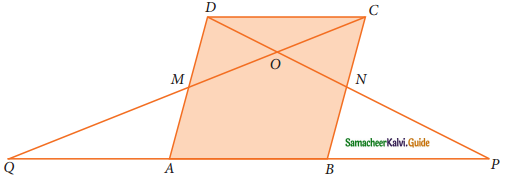Solution:
Draw OX perpendicular to QP.
In ΔADP, MN = $$\frac{1}{2}$$ AP,
In ΔBCQ, MN = $$\frac{1}{2}$$ QB
So, AP = BQ (or) AB + BP = AB + QA
∴ PB = QA
∴ QA = AB = BP (or) QP = QA + AB + BP = 3 AB
Area of ΔOQP = $$\frac{1}{2}$$ × QP × OX
= $$\frac{1}{2}$$ × 3 AB × OX
= $$\frac{3}{2}$$ × AB × OX
= $$\frac{3}{2}$$ AB (OY + YX)
= $$\frac{3}{2}$$ × AB × OY + $$\frac{3}{2}$$ × AB × YX (AB = MN)
= $$\frac{3}{2}$$ × MN × OY + $$\frac{3}{2}$$ × AB × YX
= 3 Area ΔOMN + $$\frac{3}{2}$$ + Area ΔBNM
= 3[$$\frac{1}{4}$$ area of MNCD] + $$\frac{3}{2}$$[$$\frac{1}{2}$$ area of ABCD]
= $$\frac{3}{4}$$[$$\frac{1}{2}$$ area of ABCD] + $$\frac{3}{4}$$[area of ABCD]
= $$\frac{3}{8}$$ area of ABCD + $$\frac{3}{4}$$ area of ABCD
= area of ABCD [$$\frac{3}{8}$$ + $$\frac{3}{4}$$]
= area of ABCD ($$\frac{3+6}{8}$$)
= $$\frac{9}{8}$$ area of ABCD.
Hence it is proved.